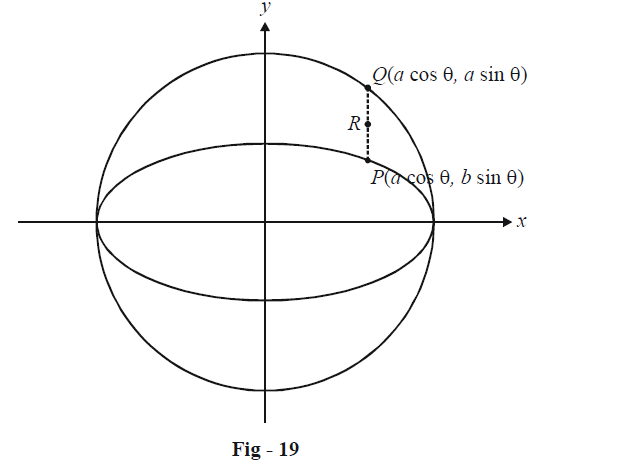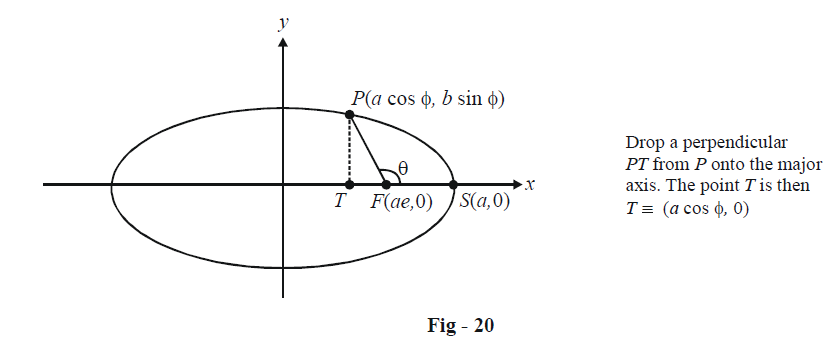# Basic Examples On Ellipses Set-5

Go back to  'Ellipse'

Example – 12

Let P be a point on the ellipse $$\frac{{{x^2}}}{{{a^2}}} + \frac{{{y^2}}}{{{b^2}}} = 1,\,\,\,\,0 < b < a.$$ Let the line parallel to the y-axis passing through P meet the circle $${x^2} + {y^2} = {a^2}$$ at the point Q such that P and Q are on the same side of the x-axis. For two positive real numbers r and s, find the locus of the point R on PQ such $$PR:RQ = r:s$$ as P varies over the ellipse.

Solution: The circle $${x^2} + {y^2} = {a^2}$$ is the auxiliary circle of the given ellipse.We can assume point P to be $$(a\cos \theta ,\,b\sin \theta )$$ so that Q will be $$(a\cos \theta ,\,b\sin \theta )$$ The point R (h, k) divides the segment PQ internally in the ratio r : s. Thus,

$h = \frac{{ar\cos \theta + as\cos \theta }}{{r + s}},\,\,k = \frac{{ar\sin \theta + bs\sin \theta }}{{r + s}}$

We need to eliminate $$\theta$$ from these two relations to obtain a relation between h and k. Thus,

$\cos \theta = \frac{h}{a},\,\,\,\,\,\sin \theta = \frac{{k(r + s)}}{{ar + bs}}$

Squaring and adding the two gives us the required relation:

$\frac{{{h^2}}}{{{a^2}}} + \frac{{{{(r + s)}^2}{k^2}}}{{{{(ar + bs)}^2}}} = 1$

The required locus of R is

$\frac{{{x^2}}}{{{a^2}}} + \frac{{{{(r + s)}^2}{y^2}}}{{{{(ar + bs)}^2}}} = 1$

which is an ellipse, as might have been expected.

Example - 13

Consider the ellipse $$\frac{{{x^2}}}{{{a^2}}} + \frac{{{y^2}}}{{{b^2}}} = 1.$$ Let F be its focus (ae, 0) and S be its vertex (a, 0). Consider any point P on the ellipse whose eccentric angle is $$\phi ,$$ while $$\angle SFP = \theta .$$ Prove that

$\tan \frac{\theta }{2} = \sqrt {\frac{{1 + e}}{{1 - e}}} \tan \left( {\frac{\phi }{2}} \right)$

Solution:We have, from the figure,

$\cos \theta = \frac{{ - FT}}{{PF}}$

Since F is the focus, note that PF will simply be e times the distance of P from the directrix $$x = \frac{a}{e}.$$ Thus,

$$PF = e\left( {\frac{a}{e} - a\cos \phi } \right)$$

\begin{align}&\qquad\qquad\;\; = a - ae\cos \phi \\&\Rightarrow \quad \cos\theta = \frac{{a(\cos \phi - e)}}{{a(1 - e\cos \phi )}} = \frac{{\cos \phi - e}}{{1 - e\cos \phi }}\qquad\qquad...\left( 1 \right)\end{align}

Thus,

\begin{align}&\qquad\quad{\tan ^2}\left( {\frac{\theta }{2}} \right) = \frac{{1 - \cos \theta }}{{1 + \cos \theta }} = \frac{{(1 + e)(1 - \cos \phi )}}{{(1 - e)(1 + \cos \phi )}}\left\{ {Using{\rm{ }}\left( 1 \right)} \right\}\\&{\rm{}} \qquad\qquad\qquad\quad= \frac{{(1 + e)}}{{(1 - e)}}{\tan ^2}\left( {\frac{\phi }{2}} \right)\\&\Rightarrow \quad\; \tan \left( {\frac{\theta }{2}} \right) = \sqrt {\frac{{1 + e}}{{1 - e}}} \tan \left( {\frac{\phi }{2}} \right)\end{align}